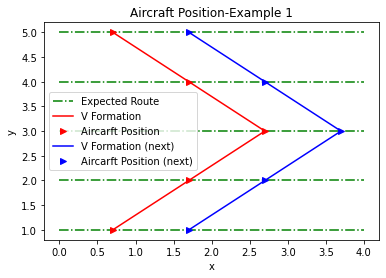## Posts

### 'V' Formation Aircraft'V' Formation Aircraft ¶ https://www.ridwanreza.com/2022/05/v-formation-aircraft.html In : import autograd.numpy as np import math from autograd import grad , jacobian , hessian import matplotlib.pyplot as plt In : # Function to calculate Steepest Descent and Newton Method def nablaf ( x , f ): j = jacobian ( f ) return j ( x ) def nabla2f ( x , f ): j = hessian ( f ) return j ( x ) def Anablaf ( x , f ): return nabla2f ( x , f ) @nablaf ( x , f ) def theta ( x , f ): return - ( nablaf ( x , f ) @nablaf ( x , f )) / ( nablaf ( x , f ) @Anablaf ( x , f )) def steepest_descent ( x , f , printt = False ): stop = False iter = 0 ; max_iter = 250 error = 0.1 ** 8 while stop == False : iter += 1 r = nablaf ( x , f ) Ar = Anablaf ( x , f ) t = theta ( x , f ) x = x + t * r #if iter%10==1 and print